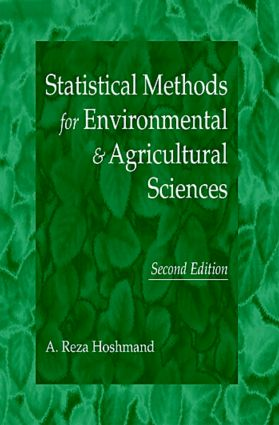# Statistical Methods for Environmental and Agricultural Sciences

## 2nd Edition

CRC Press

384 pages

Hardback: 9780849331527
pub: 1997-08-26
\$190.00
x
eBook (VitalSource) : 9780203738573
pub: 2017-11-22
from \$28.98

FREE Standard Shipping!

### Description

The first edition of this book, popular around the world, is surpassed only by this new Second Edition. Improvements such as new and revised exercises, a broad range of practical and relevant case studies, and expanded theoretical concepts make this even better for users of statistics. The book emphasizes the practical application of statistics and provides examples in various fields of environmental and agriculture sciences. Because it uses simple, non-mathematical language to present statistical techniques, the reader requires only a familiarity with elementary algebra and mathematical notations to understand and apply the concepts described.

This logically organized book covers the following topics: Part 1 introduces statistical concepts as they apply to different fields of environmental and agriculture sciences and provides descriptive measures of central tendency and variability; Part 2 covers probability and sampling concepts used in inferential statistics; Part 3 presents parametric methods in hypothesis testing, which include research designs; Part 4 discusses a number of nonparametric techniques; Part 5 explains tests of association and prediction; and lastly, analysis of change over time is detailed in Part 6. The appendices contain statistical tables for reference purposes.

### Reviews

"This valuable textbook is a useful tool in understanding how to apply and analyse statistical concepts. It is intended for students of agriculture and environmental sciences; however, other workers at agriculture and biology that are imperfectly informed about the statistical methods can use it."

-Biologia Planterum, Vol. 44, No. 2, 2001

Mathematical Symbols and Notations

General Mathematical Symbols

PART I. DESCRIPTIVE STATISTICS IN ENVIRONMENTAL AND AGRICULTURE SCIENCES

1. The What and Why of Statistics for Environmental and Agricultural Sciences

What Is Statistics?

Why Study Statistics for Environmental and Agriculture Sciences

2. Descriptive Statistical Measures

Frequency Distribution

Measures of Central Tendency

Measures of Variability

PART II. INFERENTIAL STATISTICS IN ENVIRONMENTAL AND AGRICULTURE SCIENCES

3. Probability Theory

Measurement of Probability

Optional Topic: Principles of Counting

Bayes' Theorem

Probability Distributions

4. An Introduction to Sampling Concepts

Key Sampling Concepts

Sampling Techniques in Environmental and Agriculture Sciences

Sampling Distributions

5. Estimation of Parameters: Means and Percentages

Point Estimation

Interval Estimation

Estimating Population Mean: s Known

Estimating Population Mean: s Unknown

Use of Student t Distribution

Interval Estimation of the Population Percentage

Determining Sample Size

PART III. TESTS OF COMPARISON: PARAMETRIC METHODS

6. Hypothesis Testing: One-Sample

Steps in Hypothesis Testing

Test of a Mean : Large Sample

Test of a Mean: Small Sample

Test of a Proportion

An Alternative Approach to Hypothesis Testing

Relationship between a and b Risk

7. Hypothesis Testing: Two-Sample

Two-Sample Test of Means: Large Sample

Two-Sample Test of Means: Small Sample

Two-Sample Test of Percentage: Large Sample

8. Analysis of Variance

One-Factor Analysis of Variance

Two-Factor Analysis of Variance

Latin Square Design

PART IV. NONPARAMETRIC METHODS

9. Chi-Square Analysis

Test of Differences Among Proportions

Chi-Square Test of Independence

Test of Goodness-of-Fit

Tests of Homogeneity

Sign Test

Wilcoxon Signed-Rank Test

Mann-Whitney U Test

Spearman Rank Correlation Coefficient

PART V. TEST OF ASSOCIATION AND PREDICTION

11. Simple Linear Regression and Correlation

Introduction: Bivariate Relationships

Regression Analysis

Standard Error of Estimate

Correlation Analysis

An Application Using Computer Packages

Optional Topic: Curvilinear Regression Analysis

12. Multiple Regression

Estimating Multiple Regression Equation: Least Squares Method

Standard Error of Estimate

Multiple Correlation Analysis

Interference Concerning the Regression and Correlation Coefficients

Assumptions and Problems in Multiple Linear Regression

Optional Topic: Curvilinear Multiple Regression Analysis

A Computer Application

PART VI. ANALYSIS OF CHANGE OVER TIME

13. Time Series Analysis

Secular Trend Analysis

Seasonal Variation

Identifying Cycles and Irregular Variation

14. Index Numbers

Basic Construction Techniques

Pitfalls and Some Considerations in Use of Index Numbers

APPENDICES

Binomial Distribution

Areas Under the Standard Normal Probability Distribution Between the Mean and Successive Values of z

Normal Deviates for Statistical Estimation and Hypothesis Testing

Random Digits

Critical Values of t

F Distribution

Chi-Square (c2) Distribution

Wilcoxon T Values

Critical Values of U in the Mann-Whitney Test

Values of dL and dU for the Durbin Watson Test

Index

Chapters contain Introductions, Learning Objectives, Chapter Summaries, Case Studies, and Review Questions.

Supplementary materials include a solution manual to all chapter problems, master transparencies for all figures and tables in the text, and powerpoint lecture outlines.